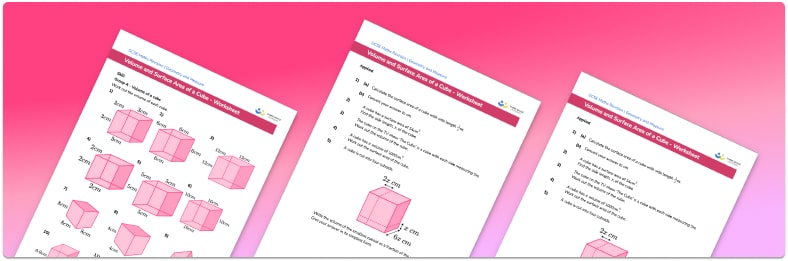# Volume and surface area of a cube worksheetHelp your students prepare for their Maths GCSE with this free volume and surface area of a cube worksheet of 30+ questions and answers

• Section 1 of the volume and surface area of a cube free worksheet contains 20+ skills-based volume and surface area of a cube questions, in 3 groups to support differentiation
• Section 2 of the printable worksheet contains 3 applied volume and surface area of a cube questions with a mix of worded problems and deeper problem solving questions
• Section 3 contains 3 foundation and higher level GCSE exam style volume and surface area of a cube questions
• Answer keys and a mark scheme for all volume and surface area of a cube questions are provided
• Questions follow variation theory with plenty of opportunities for students to work independently at their own level
• All questions created by fully qualified expert secondary maths teachers
• Suitable for GCSE maths revision for AQA, OCR and Edexcel exam boards

• This field is for validation purposes and should be left unchanged.

You can unsubscribe at any time (each email we send will contain an easy way to unsubscribe). To find out more about how we use your data, see our privacy policy.

### Volume and surface area of a cube at a glance

A cube is a 3D shape made from six identical square faces. As such, all of the side lengths of a cube are equal.

The volume of a cube is the amount of space inside the cube. The volume of a cube is found using the formula Volume = length * width * height. Volume is measured in cubic units, for example cm3.

The surface area of a cube is the sum of the areas of the faces of the cube. The surface area of a cube is found by finding the area of each face. The total surface area of the cube is the sum of these six areas. Surface area is measured in cm2.

The methods used to find the surface area and volume of cubes are the same as the methods used to find the surface area and volume of cuboids (also called rectangular prisms).

Looking forward, students can then progress to additional geometry worksheets, for example a 3D shapes worksheet or .For more teaching and learning support on Geometry our GCSE maths lessons provide step by step support for all GCSE maths concepts.

## Do you have KS4 students who need more focused attention to succeed at GCSE?There will be students in your class who require individual attention to help them succeed in their maths GCSEs. In a class of 30, it’s not always easy to provide.

Help your students feel confident with exam-style questions and the strategies they’ll need to answer them correctly with our dedicated GCSE maths revision programme.

Lessons are selected to provide support where each student needs it most, and specially-trained GCSE maths tutors adapt the pitch and pace of each lesson. This ensures a personalised revision programme that raises grades and boosts confidence.

Find out more